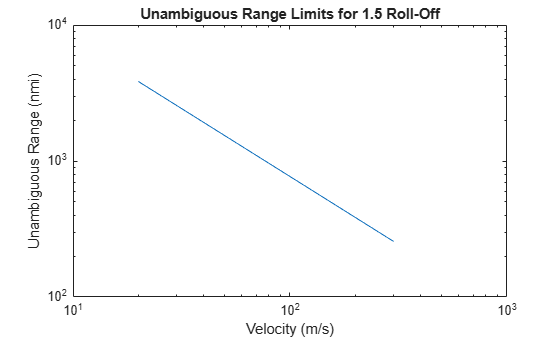# sarrange

Maximum unambiguous slant range of SAR

Since R2021a

## Syntax

``mur = sarrange(v,daz,df)``
``mur = sarrange(v,daz,df,Name,Value)``

## Description

example

````mur = sarrange(v,daz,df)` returns the maximum unambiguous slant range of a synthetic aperture radar (SAR) system.```

example

````mur = sarrange(v,daz,df,Name,Value)` specifies additional options using name-value arguments. Options include the Doppler cone angle and the antenna roll-off factor.```

## Examples

collapse all

Estimate the maximum unambiguous range of a side-looking airborne synthetic aperture radar (SAR) operating in broadside with a sensor velocity varying from 20 m/s to 300 m/s. The SAR antenna has an aperture dimension of 3 m in the azimuth direction and a transmitter that works with a 5% duty cycle. Plot the resulting unambiguous range as a function of sensor velocity.

```v = 20:10:300; daz = 3; d = 0.05;```

Compute the maximum unambiguous range in meters. Assume an antenna roll-off factor of 1.5. Convert the range to nautical miles.

```Rmet = sarrange(v,daz,d,'RollOff',1.5); Rnau = Rmet*0.00053996;```

Plot the unambiguous range as a function of the sensor velocity.

```loglog(v,Rnau) axis([10 1000 100 10000]) xlabel('Velocity (m/s)') ylabel('Unambiguous Range (nmi)') title('Unambiguous Range Limits for 1.5 Roll-Off')```## Input Arguments

collapse all

Sensor velocity in meters per second, specified as a positive real scalar or vector.

Data Types: `double`

Antenna width in the azimuth direction in meters, specified as a positive real scalar.

Data Types: `double`

Duty factor, specified as a positive real scalar in the range [`0`, `1`] or a vector. The duty factor is defined as the ratio of the pulse width to the pulse period.

Data Types: `double`

### Name-Value Arguments

Specify optional pairs of arguments as `Name1=Value1,...,NameN=ValueN`, where `Name` is the argument name and `Value` is the corresponding value. Name-value arguments must appear after other arguments, but the order of the pairs does not matter.

Before R2021a, use commas to separate each name and value, and enclose `Name` in quotes.

Example: `'RollOff',1.2,'ConeAngle',120`

Doppler cone angle in degrees, specified as a scalar in the range [`0`, `180`]. This argument identifies the direction toward the scene relative to the direction of motion of the array.

Data Types: `double`

Antenna roll-off factor, specified as a positive real scalar. This argument provides a safety factor that prevents mainlobe returns from aliasing in the pulse repetition frequency (PRF) time interval. Adjust the roll-off factor to make the PRF greater than the mainlobe Doppler bandwidth.

Data Types: `double`

## Output Arguments

collapse all

Maximum unambiguous range, returned as a matrix. The rows of `mur` correspond to the velocity values in `v`. The columns of `mur` correspond to the duty factor values in `df`.

## Version History

Introduced in R2021a